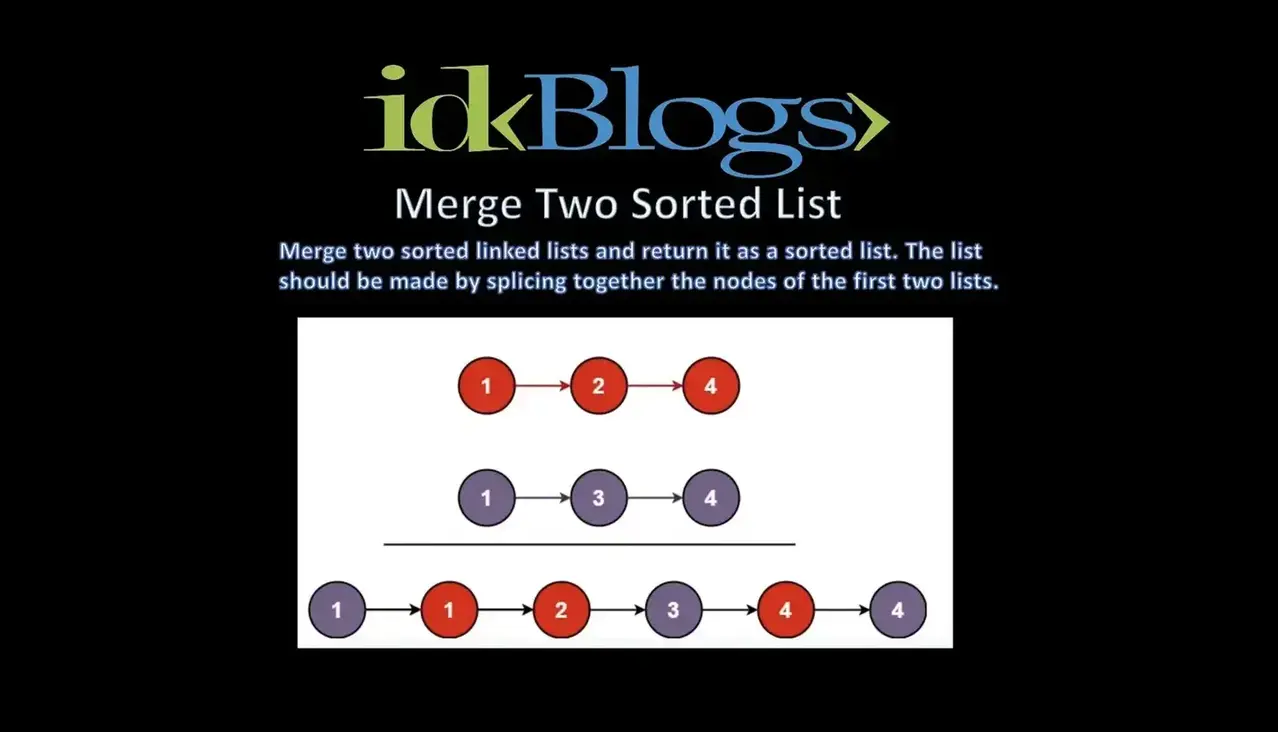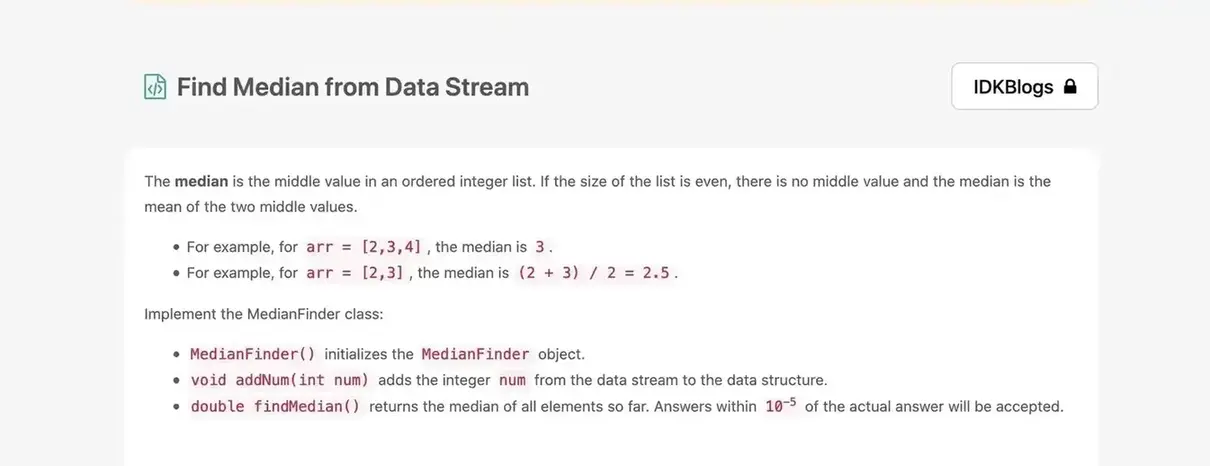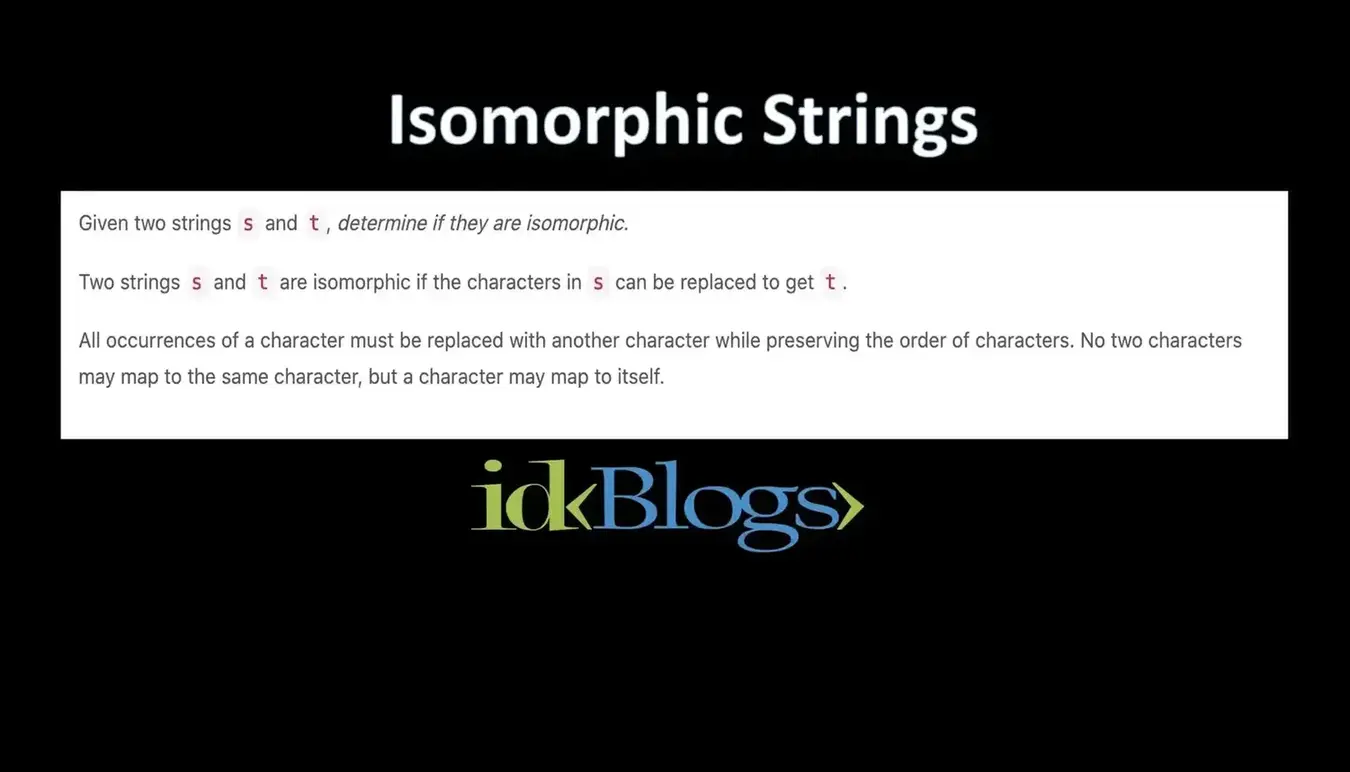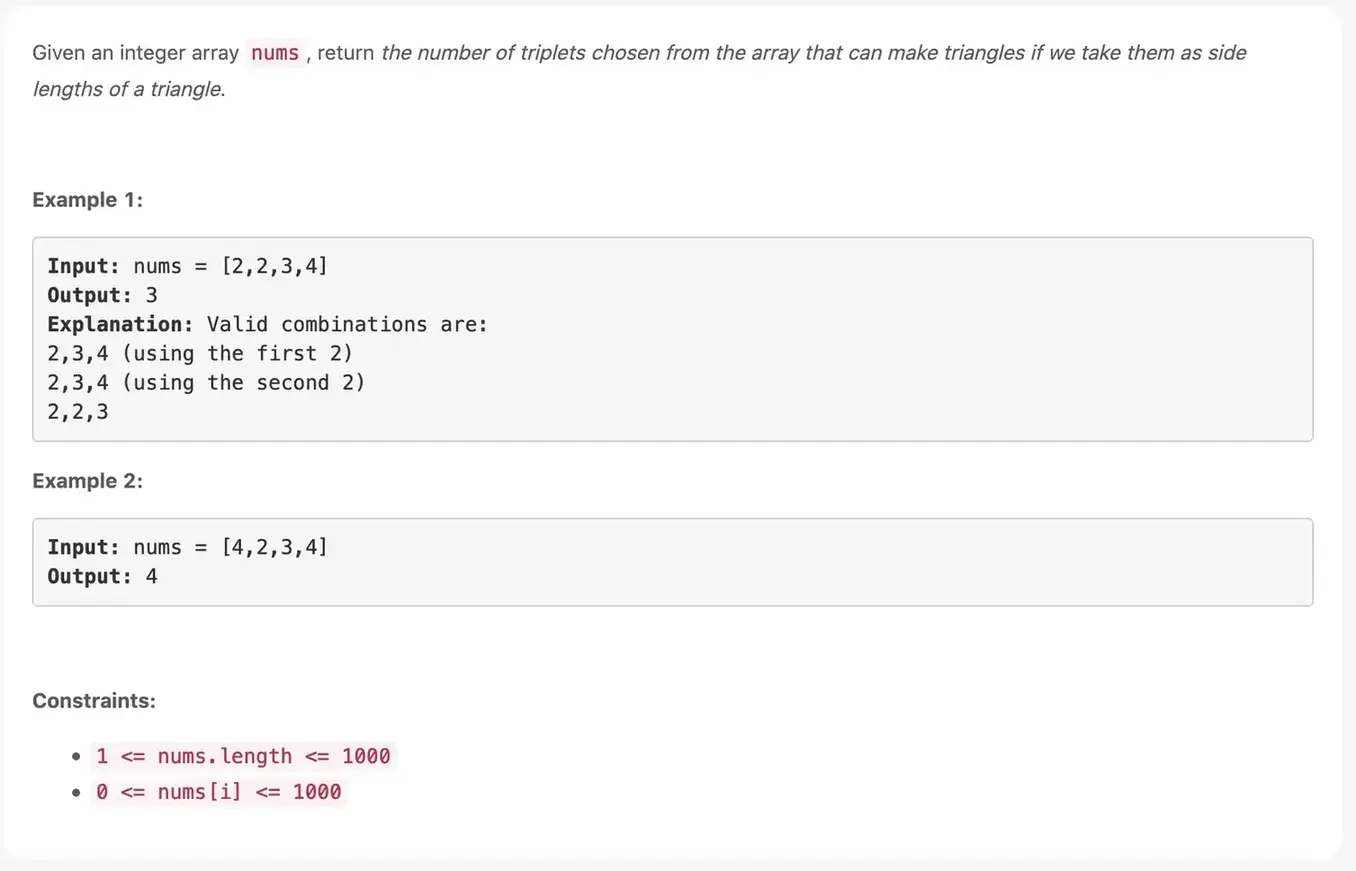# Maximum Length of Repeated Subarray

### Given two integer arrays nums1 and nums2, return the maximum length of a subarray that appears in both arrays.

idkblogs.com      July 7, 2021Shubham Verma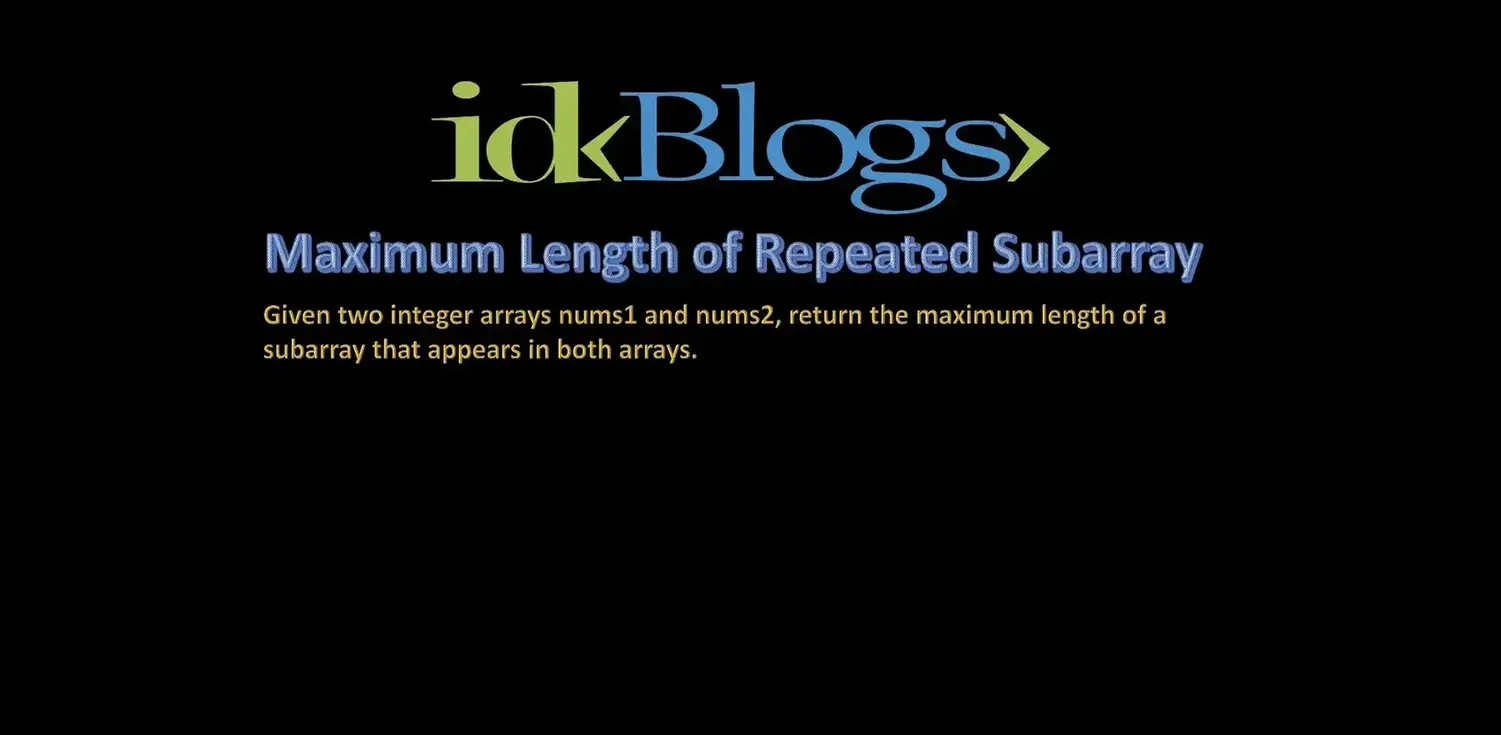# Given two integer arrays nums1 and nums2, return the maximum length of a subarray that appears in both arrays.

#### Example 1:

Input: nums1 = [1,2,3,2,1], nums2 = [3,2,1,4,7]
Output: 3
Explanation: The repeated subarray with maximum length is [3,2,1].

#### Example 2:

Input: nums1 = [0,0,0,0,0], nums2 = [0,0,0,0,0]
Output: 5

#### Constraints:

1 <= nums1.length, nums2.length <=1000
0 <= nums1[i], nums2[i] <=100

# Solution:

``````                                    var findLength = function(A,B) {

let n=A.length;
let m=B.length

let dp = new Array(n + 1);
for (let i = 0; i <= n; i++)
{
dp[i]=new Array(m+1);
for (let j = 0; j <= m; j++)
dp[i][j] = 0;
}

for (let i = n - 1; i >= 0; i--)
{
for (let j = m - 1; j >= 0; j--)
{
if (A[i] == B[j]){
let xxx=dp[j + 1][i + 1];
if(!xxx){
xxx=0;
}
dp[j][i] = xxx + 1;
}
}
}
let maxm = 0;
for (let i = 0; i < n; i++)
{
for (let j = 0; j < m; j++)
{
maxm = Math.max(maxm, dp[i][j]);
}
}

return maxm;
};

``````

# Output:Given two integer arrays nums1 and nums2, return the maximum length of a subarray that appears in both arrays.

Thank you

To read more about the technologies, Please subscribe us, You'll get the monthly newsletter having all the published article of the last month.

### OTHER POSTS